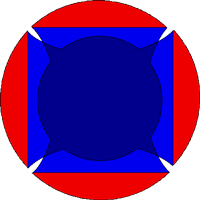Spin of the neutronSpin of the neutron

We suppose that the neutron turns around an axis (X for example). The contribution of the X-bubble to the magnetic moment will be zero.

If the negative charge radius is 1.3205 fm
and if the positive charge radius is 0.86755fm,

The contribution of the Y-bubble and the Z-bubble to the negative charge is
2*(-2e/6).

The contribution of the Y-bubble and the Z-bubble to the positive charge is
(0.86755 / 1.3205) * (2*(+1e/3)).
The contribution of the Y-bubble and the Z-bubble to the cinetic moment is
2*(+1*ħ/3 ).
The relativistic half-mass (for the negative fraction) of bubbles is (Mn/2π)/2.
The relativistic half-mass (for the positive fraction) of bubbles is (Mn/2π)/2.

The magnetic moment of the neutron is:
MMN =
(negative charge * cinetic moment * spin) / (half-mass of a neutron bubble)
+
(positive charge * cinetic moment * spin) / (half-mass of a neutron bubble)

MMN =
( ((-2e/3) * (+2ħ/3)) / (2*(Mn/4π)) ) +
( ((0.86755 / 1.3205)*(+2e/3) * (+2ħ/3)) / (2*(Mn/4π)) )
= - 1.91572 * (eħ/2Mn)
= -1,91304
* (eħ/2Mp)

with Mn = 1,0014 Mp

The experimental value is - 1.91304 * µN

(W.-M. Yao, et al., J. Phys. G 33 (2006))

The radius of charge of the proton is :
0.8750 fm (±0.0068) (Biraben et al. ; 2007)

The radius of charge of the proton in the muonic hydrogen is:
0.8418 (±0.0007) (Pohl et al. ; 2011)

 Copyright : Benoît PRIEUR; Contact: bubblestheory at gmail.com